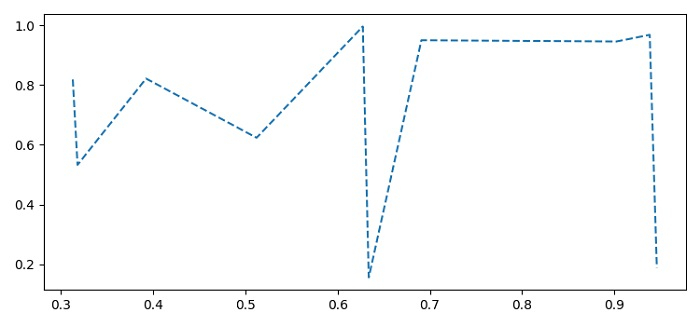# How to plot a dashed line on a Seaborn lineplot in Matplotlib?

To plot a dashed line on a Seaborn lineplot, we can use linestyle="dashed" in the argument of lineplot().

## Steps

• Set the figure size and adjust the padding between and around the subplots.

• Create x and y data points using numpy.

• Use lineplot() method with x and y data points in the argument and linestyle="dashed".

• To display the figure, use show() method.

## Example

import seaborn as sns
import numpy as np
from matplotlib import pyplot as plt
plt.rcParams["figure.figsize"] = [7.50, 3.50]
plt.rcParams["figure.autolayout"] = True
x = np.random.rand(10)
y = np.random.rand(10)
ax = sns.lineplot(x=x, y=y, linestyle="dashed")
plt.show()

## OutputUpdated on: 01-Jun-2021

4K+ Views